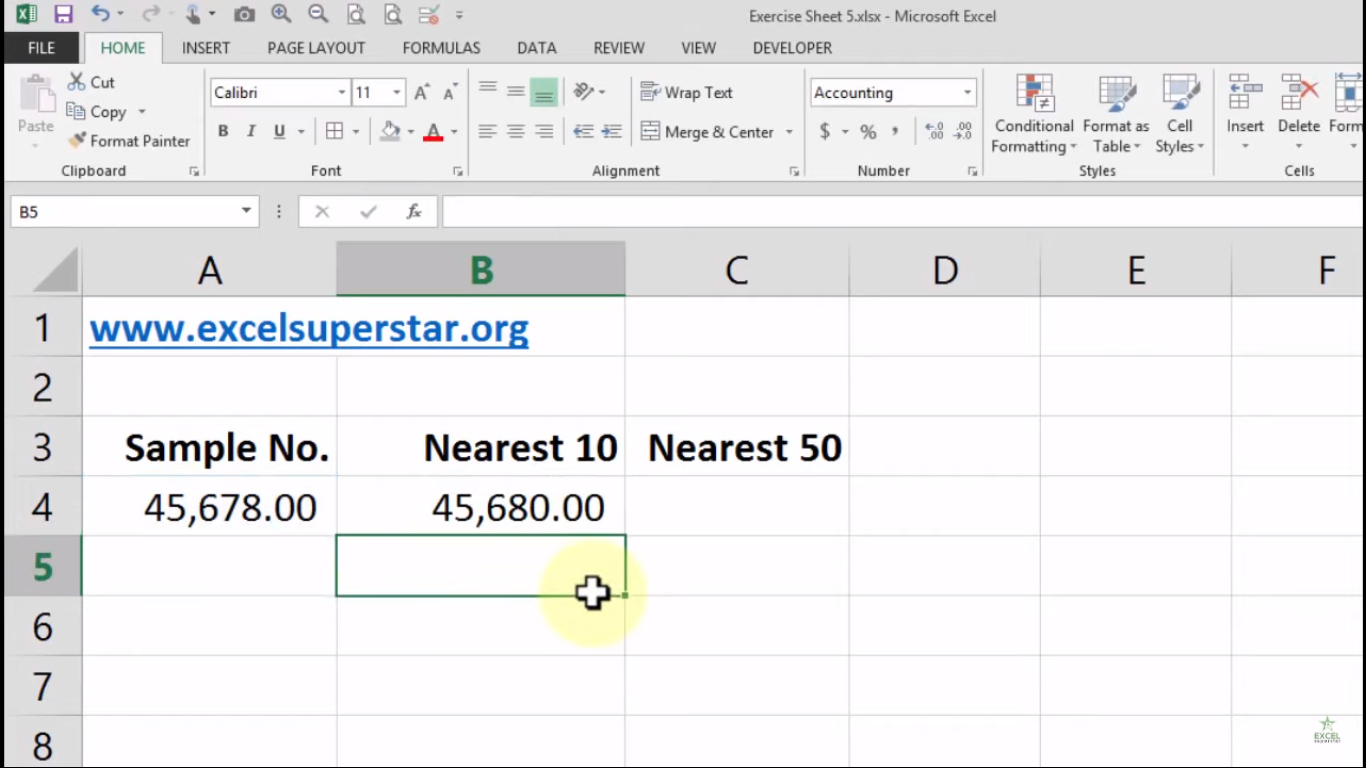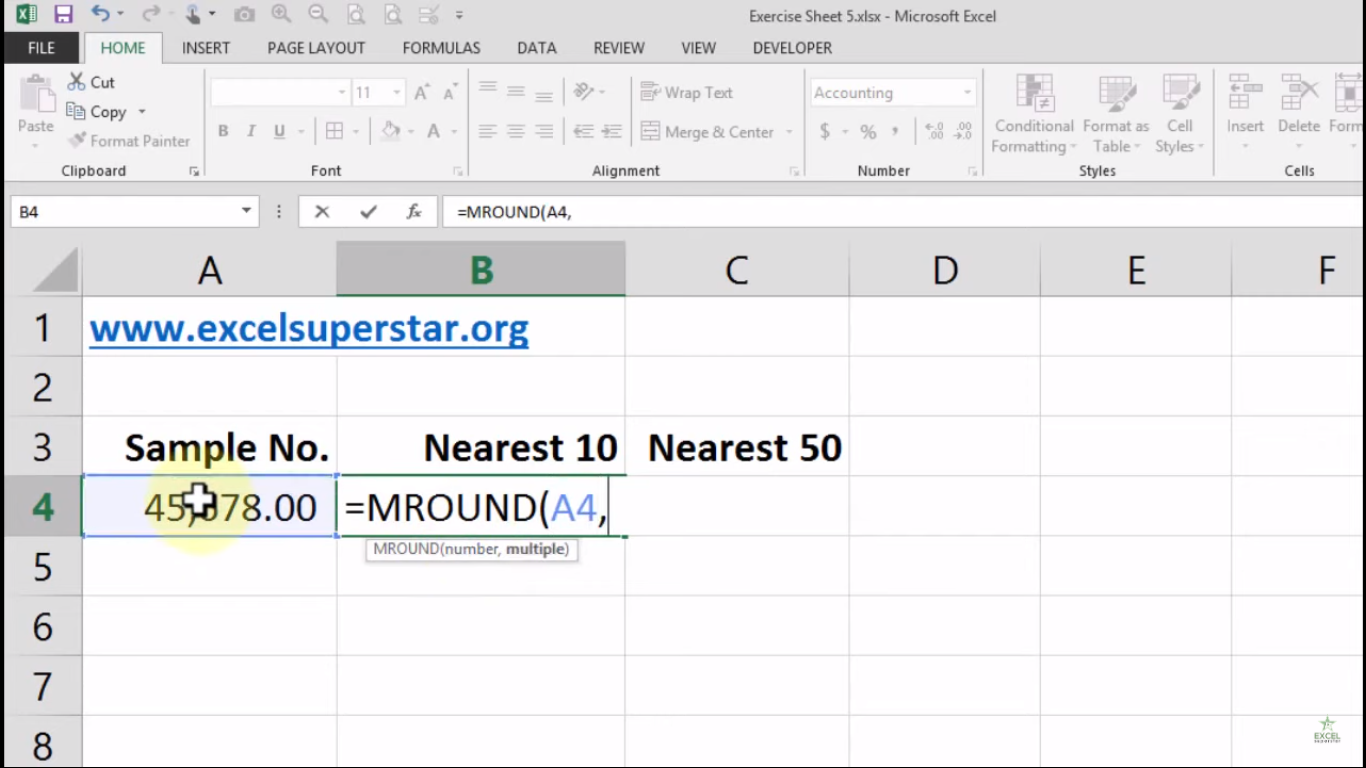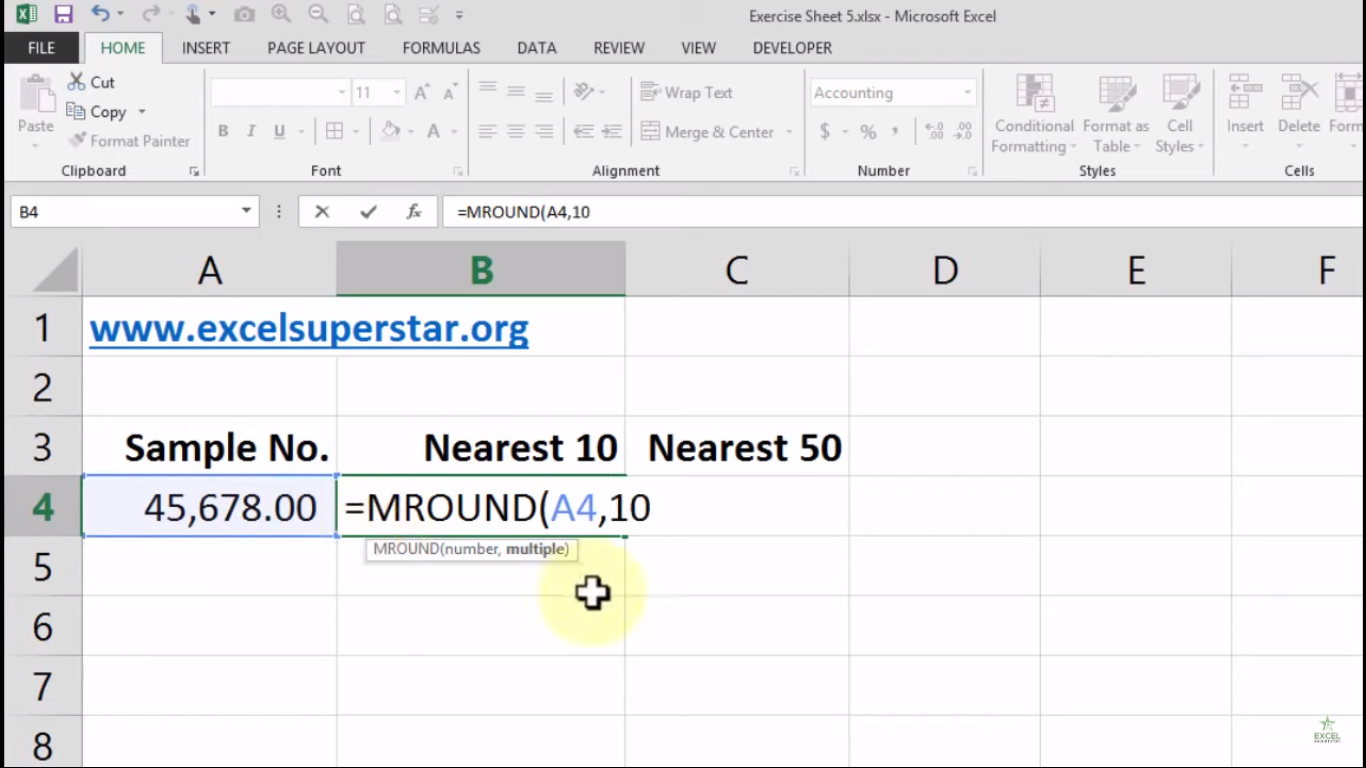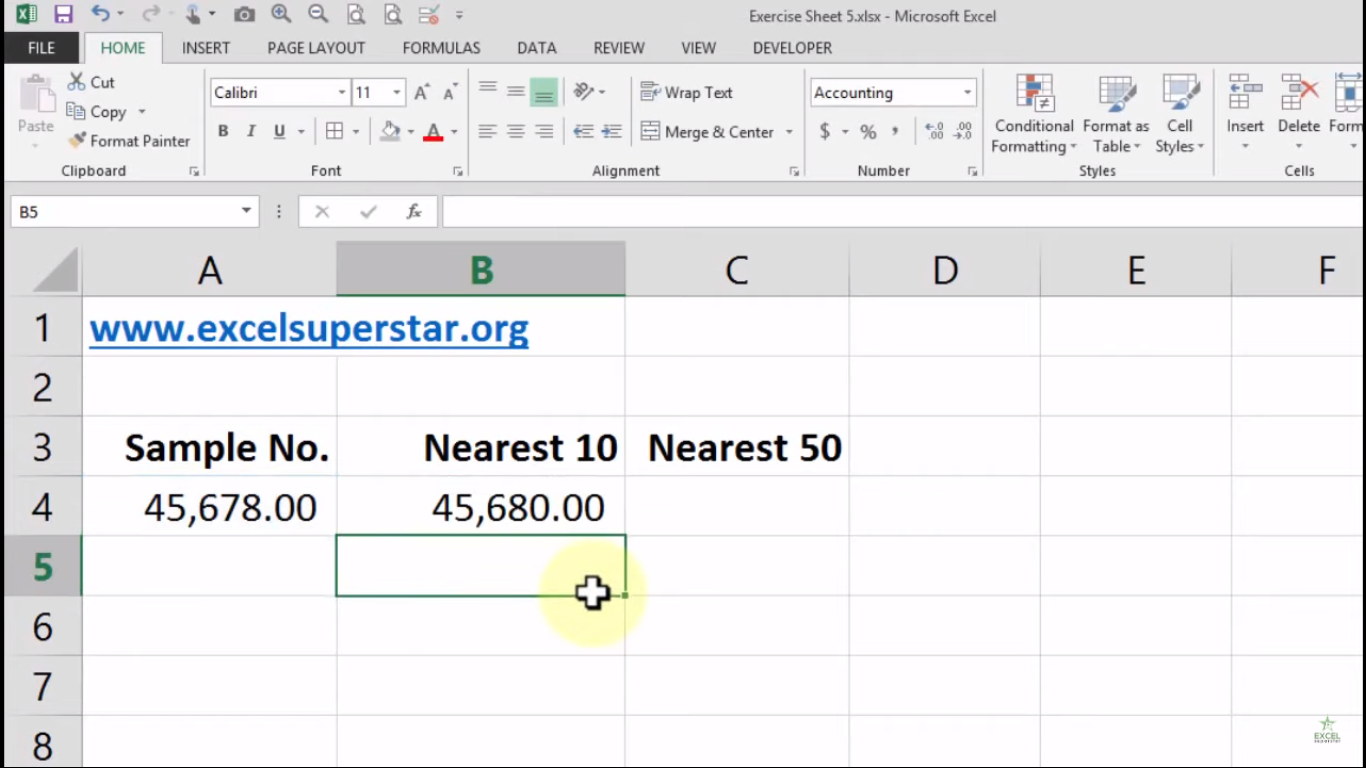# Excel MROUND FunctionIn this tutorial, we will learn about How to use MROUND Function in Excel

Example:

There is a sample number 45,678.00, which we want to round the number to Nearest 10 means the answer should be 45,680.00, and the last number should remain 0

To round a number in to the nearest 5, you can use MROUND Function like this:

1. Write = mround and press the Tab KeyThe MROUND function syntax has the following arguments:

1. Number – The number that should be rounded.

2. Multiple – The multiple to use when rounding.

2. Select the number to be rounded3. Write 10 as the multiple to use for rounding4. Close the bracket and press Enter5. Excel will calculate using the MROUND Function